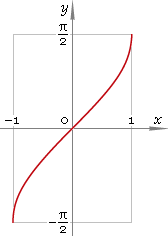The Art of Mathematics

# arcsin — trigonometric arc sine function

## 1. Definition

Arc sine is inverse of the sine function.

arcsinxsininvx

## 2. Graph

Arc sine is monotone antisymmetric function defined in the range [−1, 1]. Its graph is depicted below in fig. 1.Fig. 1. Graph of the arc sine function y = arcsinx.

Function codomain is limited to the range [−π/2, π/2].

## 3. Identities

Complementary angle:

arcsinx + arccosx = π/2

and as consequence:

arcsin cos φ = π/2 − φ

Negative argument:

arcsin(−x) = −arcsinx

Reciprocal argument:

arsin(1/x) = arccscx

Sum and difference:

arcsinx + arcsiny = arcsin[x√(1 − y2) + y√(1 − x2)]
arcsinx − arcsiny = arcsin[x√(1 − y2) − y√(1 − x2)]

Some argument values:

Argument xValue arcsinx
00
(√6 − √2) /4π/12
(√5 − 1) /4π/10
√(2 − √2) /2π/8
1 /2π/6
√(10 − 2√5) /4π/5
1 /√2π/4
(√5 + 1) /43π/10
√3 /2π/3
√(2 + √2) /23π/8
√(10 + 2√5) /42π/5
(√6 + √2) /45π/12
1π/2
Table 1. Arc sine for some argument values.

## 4. Derivative and indefinite integral

Arc sine derivative:

arcsin′x = 1 /√(1 − x2)

Indefinite integral of the arc sine:

∫ arcsinx dx = x arcsinx + √(1 − x2) + C

where C is an arbitrary constant.

## 5. How to use

To calculate arc sine of the number:

``arcsin(−1);``

To get arc sine of the complex number:

``arcsin(−1+i);``

To get arc sine of the current result:

``arcsin(rslt);``

To get arc sine of the number z in calculator memory:

``arcsin(mem[z]);``

## 6. Support

Trigonometric arc sine of the real argument is supported in free version of the Librow calculator.

Trigonometric arc sine of the complex argument is supported in professional version of the Librow calculator.